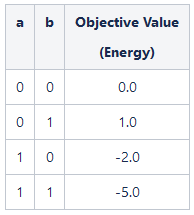# SampleSet energy

Hey everyone, how does dwave calculated sampleSet energy? I know that when we sample using (say) sample_ising(), we get a sampleSet object with: samples, energy of each sample and number of occurrences. My question is how does dwave find the energy associated with a particular sample?

I couldn't find many references on this online. Thanks!

1 comment
• Hi Sehmimul,

The energy landscape is defined by the linear and quadratic biases of a problem formulation.

For example, consider an example where the objective function is -2a+b-4ab and a,b are binary variables. The solution space for this example is:Submitting this problem to the QPU:

`>>> from dwave.system import DWaveSampler, EmbeddingComposite>>> sampler = EmbeddingComposite(DWaveSampler())>>> Q = {('a', 'a'): -2, ('b', 'b'): 1, ('a','b'): -4}>>> sampleset = sampler.sample_qubo(Q, num_reads = 10)>>> print(sampleset)   a  b energy num_oc. chain_.0  1  1   -5.0      10     0.0['BINARY', 1 rows, 10 samples, 2 variables]`

As expected, the example above returns the lowest energy solution with a=b=1 and energy -5.0.

You might also find our getting started guide helpful: https://docs.dwavesys.com/docs/latest/doc_getting_started.html